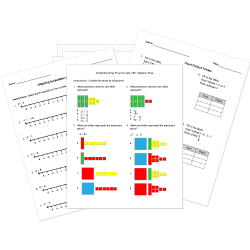Looking for Algebra worksheets?
Check out our pre-made Algebra worksheets!
 Tweet##### Browse Questions
• Arts (4578)
• English Language Arts (62416)
• English as a Second Language ESL (44804)
• Health and Medicine (9413)
• Life Skills (2966)
• Math (31121)

• ### Vectors

• #### Trigonometry

• Physical Education (4157)
• Science (53956)
• Social Studies (30305)
• Study Skills and Strategies (382)
• Technology (2641)
• Vocational Education (7171)

# Nonlinear Equations and Functions Questions - All Grades

You can create printable tests and worksheets from these Nonlinear Equations and Functions questions! Select one or more questions using the checkboxes above each question. Then click the add selected questions to a test button before moving to another page.

Previous Next
Grade 10 Nonlinear Equations and Functions CCSS: HSA-REI.A.2
Solve for $y$.
$(20y-12)/(4y)=4$
1. $3$
2. $5$
3. $2$
4. $-1$
Grade 10 Nonlinear Equations and Functions CCSS: HSA-REI.A.2
Solve for $t$.
$sqrt(5t+11)=6$
1. $11$
2. $46/55$
3. $10$
4. $5$
Grade 10 Nonlinear Equations and Functions CCSS: HSA-REI.A.2
Grade 10 Nonlinear Equations and Functions
Grade 11 Nonlinear Equations and Functions CCSS: HSA-REI.A.2
Solve for all possible values of $x$. $sqrt(x^2+4x-16)=4$
1. No Solution
2. $-8, 4$
3. All real numbers
4. $-4, 16$
Grade 11 Nonlinear Equations and Functions CCSS: HSA-REI.A.2
Grade 11 Nonlinear Equations and Functions CCSS: HSA-REI.A.2
Grade 9 Nonlinear Equations and Functions
In the year 2000, the population of Virginia was about 7,400,000. Between the years 2000 and 2004, the population in Virginia grew at a rate of 5.4%. At this growth rate, what function gives the population x years after 2000?
1. $f(x) = 7,400,000 (1 + .054)^x$
2. $f(x) = 7,400,000 (1 - .054)^x$
3. $f(x) = 7,400,000 (.054)^x$
4. $f(x) = 7,400,000x^(.054)$
Grade 9 Nonlinear Equations and Functions CCSS: HSA-REI.A.2
Grade 10 Nonlinear Equations and Functions CCSS: HSA-REI.A.2
Solve for $x$.
$(2x-3)/(x-5)=3$
1. $12$
2. $21/7$
3. $2$
4. $2/3$
Grade 9 Nonlinear Equations and Functions CCSS: HSA-REI.A.2
Solve the equation $5/(2x-3)=3/(x+5)$.
1. $x=16$
2. $x=4$
3. $x=19$
4. $x=34$
Grade 12 Nonlinear Equations and Functions
Grade 10 Nonlinear Equations and Functions CCSS: HSA-REI.A.2
Solve for $x$.
$sqrt(7x)/x=x/7$
1. $1$
2. $1/7$
3. $7$
4. $-1$
Grade 11 Nonlinear Equations and Functions CCSS: HSG-GPE.A.3

This question is a part of a group with common instructions. View group »

After some more algebra, the equation becomes:
$(c^2-a^2) x^2 - y^2 = (c^2 - a^2) y^2$
What happened to the plus-minus sign that was in the original equation in the seventh question?
1. Since there are no more square root terms, it is unnecessary.
2. Each term with it was eventually squared, and a squared term must be positive it.
3. Because the terms were moved from one side to another multiple times, it is no longer necessary.
4. Any terms with it canceled out.
Grade 11 Nonlinear Equations and Functions CCSS: HSG-GPE.A.3

This question is a part of a group with common instructions. View group »

After some more algebra, and then moving all terms with $x$ or $y$ to the left side of the equation (and all others to the right), the resulting equation is:

 $a^2 x^2 - c^2 x^2 + a^2 y^2$ $=$ $a^4 -a^2 c^2$ $(a^2 - c^2)x^2 + a^2 y^2$ $=$ $(a^2 - c^2) a^2$ $b^2 x^2 + a^2 y^2$ $=$ $a^2 b^2$ $x^2 / a^2 + y^2 / b^2$ $=$ $1$

This is the formula for the equation of an ellipse centered at $(0,0)$. Why can the substitution $b^2 = a^2-c^2$ be made?
1. Since $b$ is undefined so far, it can be defined as any value. Then, using the Pythagorean theorem, $a^2 + b^2 = c^2$, simply rearrange to solve for $b^2$.
2. Knowing that the semi-minor axis is $b$ units long, one can substitute the square of this value for $a^2-c^2$.
3. Since $b$ is not yet defined, it can be used to simplify the equation by defining $b^2 = a^2 - c^2$. A positive value for $a^2-c^2$ exists since $|a| > |c|$.
4. Projecting $b$, the length of the semi-minor axis, onto the semi-major axis it is seen that $b=a-c$. Then, simply square both sides of the equation.
Grade 9 Nonlinear Equations and Functions
$4x^3 = - 1/54$
1. $- 1/3$
2. $- 1/6$
3. $- 1/9$
4. $- 1/12$
Grade 9 Nonlinear Equations and Functions CCSS: HSA-REI.A.2
Grade 10 Nonlinear Equations and Functions
If $4x^3 = - 1/54, x =$
1. $- 1/3$
2. $- 1/6$
3. $- 1/9$
4. $- 1/12$
Grade 11 Nonlinear Equations and Functions CCSS: HSG-GPE.A.3

This question is a part of a group with common instructions. View group »

Substituting the equations for $d_1, d_2$ found in the second and third questions into the above equation, and then moving the second square root term to the right side of the equation results in the following:
$sqrt( (x+c)^2 + y^2) = 2a - sqrt( (x-c)^2 + y^2)$
The next step will be to square both sides. Why was the second square root term moved to the right side of the equation before squaring, instead of just squaring both sides immediately?
1. It gets rid of all square root terms immediately.
2. It will make the algebra easier later on, since the two square root terms are not multiplied together.
3. This ensures that there won't be any multiple answers that usually result from square roots (taking the positive and negative).
4. This makes that equation more balanced, which looks nicer.
Previous Next
You need to have at least 5 reputation to vote a question down. Learn How To Earn Badges.